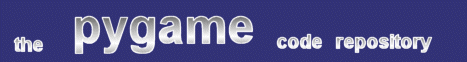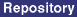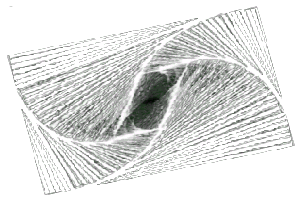### Title: 'Selfrep' Effect Script

Author: Sebastian "WhiteTiger" John (TheWhiteTiger at gmx.net)
Submission date: September 27, 2002

Description: Three different experiments with self-representation.pygame version required: Any
SDL version required: Any
Python version required: Any

Comments: Self-representation is produced when an effect's output is turned into its input - under these conditions, recursion results, and small changes can have dramatic results. Mr. John demonstrates this with the following script, which has three 'flavors' - use the 1, 2, and 3 keys to cycle through them.

```
"""
Name:		Selfrep
Author:		Sebastian "WhiteTiger" John
Email:		TheWhiteTiger@gmx.net

This is public domain code.

This effect (my first one!!! ;) does nothing more than take a surface (in
this example a rectangle), rotate it, scale it down, and put the rectangle
around it again, and then draw it... The three different transform functions
in this file should do almost the same, but they look quite a bit different.

I got the idea for this effect from 'Gödel, Escher, Bach' by Douglas R.
Hofstadter, in one chapter he wrote about self-replication, with the example
of a video camera directly connected to a TV, and then have the camera
capture the TV itself... I wanted to get this with my computer, too, but it
seems to be a lot harder than I thought... This effect is not what I really
wanted, but it's nice, too... :)

Keyboard controls:
"1" => transform effect one
"2" => transform effect two
"3" => transform effect three
"q" => quit (also working: mouse click, Escape)
"""

import pygame
from pygame.locals import *

# screen resolution
RES = RESX, RESY = (300, 200)
SCR_RECT = (0, 0, RESX, RESY)

# configuration flags
INITIAL_RECT = 1
RESET_ANGLE = 0

# drawing color... you could also change it to 'COLOR = (255,0,0)'...
COLOR = (255, 255, 255)

# rotozoom parameters
ANGLE = 0
SCALE = 0.94
# or .8, .9, .92, .95, ...

def inc_angle(step=1):
global ANGLE
ANGLE += step
if ANGLE > 360: ANGLE -= 360

def transform1(surf):
# 0.92 looks quite better than the default of 0.94...
surf = pygame.transform.rotozoom(surf, 5, 0.92)
pygame.draw.rect(surf, COLOR, SCR_RECT, 10)
return surf

def transform2(surf):
inc_angle()
surf = pygame.transform.rotozoom(surf, ANGLE, SCALE)
newsurf = pygame.Surface(RES)
x, y = (RESX-RESX*SCALE)/2, (RESY-RESY*SCALE)/2
newsurf.blit(surf, (x, y))
pygame.draw.rect(newsurf, COLOR, SCR_RECT, 10)
return newsurf

def transform3(surf):
inc_angle()
surf = pygame.transform.rotozoom(surf, ANGLE, SCALE)
surf = pygame.transform.scale(surf, RES)
pygame.draw.rect(surf, COLOR, SCR_RECT, 10)
return surf

def init_surface():
surf = pygame.Surface(RES)
if INITIAL_RECT:
pygame.draw.rect(surf, COLOR, SCR_RECT, 10)
return surf

def new_transform(key):
if key == K_1:
transform = transform1
elif key == K_2:
transform = transform2
elif key == K_3:
transform = transform3

if RESET_ANGLE:
global ANGLE
ANGLE = 0

surf = init_surface()

return transform, surf

def main():
# initialize the screen
pygame.init()
screen = pygame.display.set_mode(RES)

# initial transform function and surface
transform_surface = transform3
surf = init_surface()

done = 0
while not done:
# keep an eye on the events
for ev in pygame.event.get():
if ev.type in (QUIT, MOUSEBUTTONDOWN):
done = 1
elif ev.type == KEYDOWN:
if ev.key in (K_ESCAPE, K_q):
done = 1
elif ev.key in (K_1, K_2, K_3):
transform_surface, surf = new_transform(ev.key)

# do all the nice stuff with the surface
surf = transform_surface(surf)

# draw it
screen.blit(surf, (0, 0))
pygame.display.flip()
pygame.time.wait(50)

if __name__ == "__main__":
main()

```
 From: Anonymous Date: October 18, 2002 03:24 GMT This is actually close to a special case of an iterated function system - a mathematical formalism used to generate some types of fractals. The (a) formal definition is a set of transforms of the form (in 2D) t[i] (x scale, y scale, x translate, y translate, x rotate, y rotate) We denote a set of t[i] by T. To generate the (often fractal) set, you apply T to a region and then to each of the subregions that contain the images of T, and so on... It is also interesting to give each of the t[i] a probability of being applied at any given iteration. Though this converges to the same set as the deterministic system, it looks fuzzier along the way. Try http://classes.yale.edu/fractals/ for a clearer and more error-free explanation.

 Feedback